Latest Banking jobs   »   Quantitative Aptitude Quiz For IBPS Clerk...

# Quantitative Aptitude Quiz For IBPS Clerk Prelims 2021- 6th August

Directions (1-5): Each of the following questions is provided with 2 statements i.e. Statement I & Statement II. You have to study them and find which statement (s) is/are necessary to answer the question as per the instruction set given below.
(a) Only Statement I is sufficient to give the answer of the question
(b) Only Statement II is sufficient to give the answer of the question
(c) Neither Statement I nor Statement II is sufficient to give the answer of the question
(d) Either Statement I or Statement II is sufficient to give the answer of the question
(e) Both Statement I & Statement II is sufficient to give the answer of the question

Q1. What is value of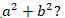Statement I: 50% of a is 25% of b.
Statement II: 30% of a is 15.

Q2. How many cars are there in the parking?
Statement I: if 10 cars more is parked, it will be twice of actual cars parked.
Statement II: there is a space for 10 more cars.

Q3. What is average age of P, Q & R?
Statement I: average age of P and R is 15 years.
Statement II: Q is 4 years younger to P.

Q4. What is speed of train? (in kmph)
Statement I: The train crosses a man standing at platform in 10 sec & it’s length is 100 m.
Statement II: The train covers a journey of 200 kms in 4 hours.

Q5. What is area of circle?
Statement I: the perimeter of circle is equal to perimeter of square.
Statement II: the perimeter of semicircle of same radius as of circle is 36 cm.

Directions (6-7): Each of the following questions is provided with 2 statements i.e. Statement I & Statement II. You have to study them and find which statement (s) is/are necessary to answer the question as per the instruction set given below.
(a) Only Statement I is necessary
(b) Either Statement I or Statement II is sufficient to answer
(c) Both Statement I & Statement II are necessary to answer
(d) Only Statement II is necessary
(e) Neither Statement I nor Statement II is sufficient to answer

Q6. What is speed of boat in still water?
Statement I: the speed of boat in downstream is 20 kmph.
Statement II: the upstream speed is 12 kmph.

Q7. How many blue balls are in the bag?
Statement I: there are 20 balls in the bag of which red balls are 5.
Statement II: the probability of drawing a blue ball from bag having 20 balls is 0.25.

Directions (8-12): In the given questions, two quantities are given, one as Quantity I and another as Quantity II. You have to determine relationship between two quantities and choose the appropriate option.
(a) Quantity I > Quantity II
(b) Quantity I < Quantity II
(c) Quantity I ≥ Quantity II
(d) Quantity I ≤ Quantity II
(e) Quantity I = Quantity II or no relation

Q8. 7x men can complete a piece of work in x/4 days while 3y men can complete the same work in 3y/7 days.
Quantity I: Value of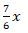Quantity II: Value of y + 5

Q9. Quantity I : Sum of first 50 odd natural numbers.
Quantity II : sum of first 50 even natural numbers.

Q10. Quantity I: A can do a piece of work in 4 days and B can do the same piece of work in 6 days. C is 20 % more efficient than B, no. of days to complete the work when all work together.
Quantity II: P can do a piece of work in 15 days while Q can do the same piece of work in 22 days, working together no. of days required by P and Q to complete the work.

Q11. Quantity I (in km/hr.): A train crosses a pole in 8 seconds and a 200m long platform in 28 seconds. What is speed of the train? (km/hr)
Quantity II (in days): P can complete a work in 45 days and P and Q together can complete the same work in 18 days. In how many days Q alone will do the same work?

Q12. Quantity I (in cm): area of rectangle is 270cm² and difference between length and breadth is 3cm. Find the sum of length and breadth?
Quantity II (y): y²-31y – 66 = 0

Directions (13-15): What should come in place of question mark (?) in the following questions?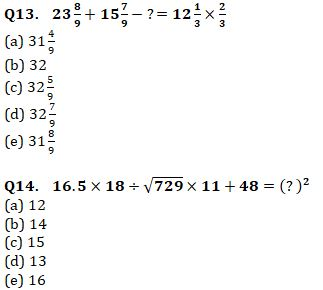Q15. 4444 ÷ 44 + 3003 ÷ 3 =? + 841
(a) 271
(b) 261
(c) 251
(d) 281
(e) 291

Solution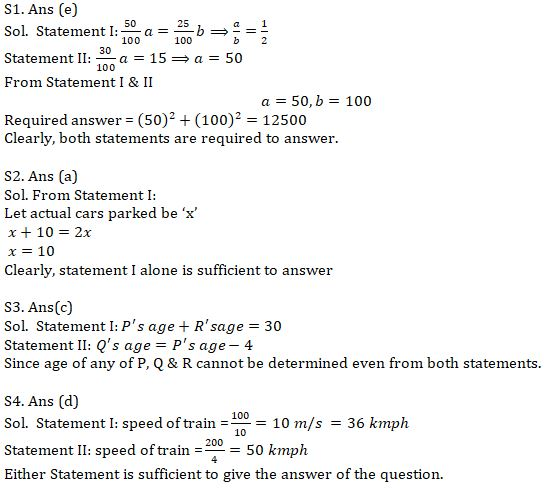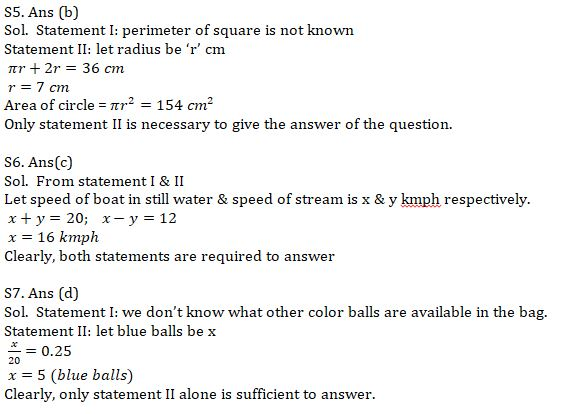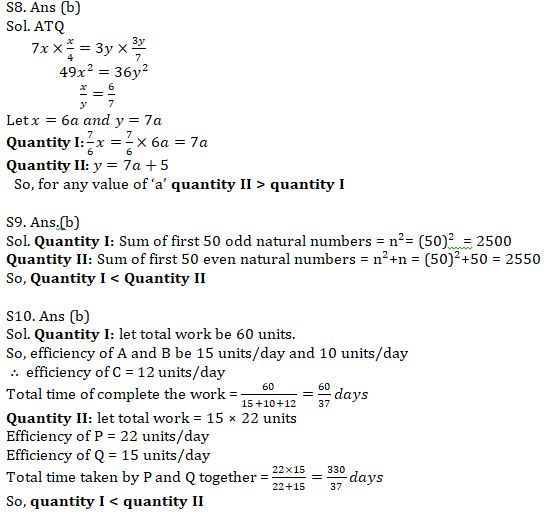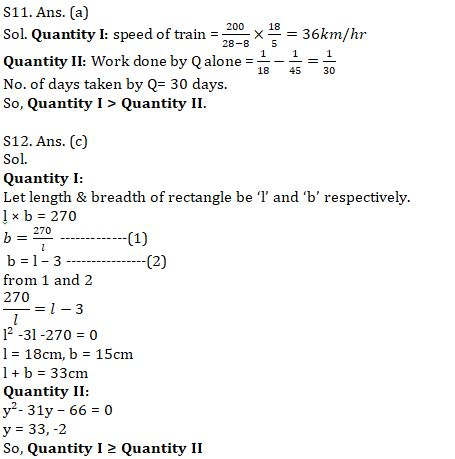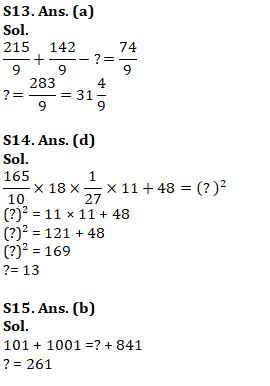#### Congratulations!Incorrect details? Fill the form again here

•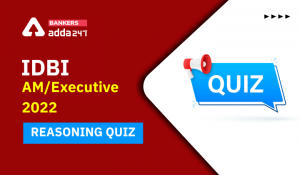Reasoning Ability Quiz For IDBI AM/Execu...
•Reasoning Ability Quiz For IDBI AM/Execu...
•Quantitative Aptitude Quiz For IDBI AM/E...
•Quantitative Aptitude Quiz For IDBI AM/E...
•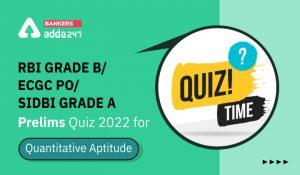Quantitative Aptitude Quiz For RBI Grade...
•Quantitative Aptitude Quiz For SBI Clerk...